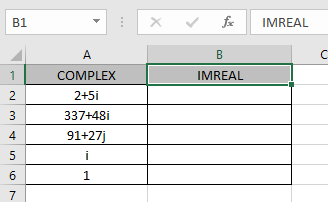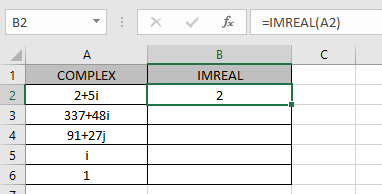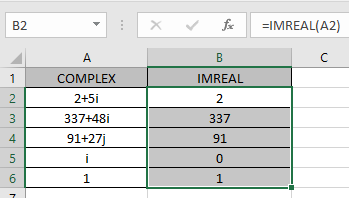# How to use the IMREAL Function in Excel

In this article, we will learn about how to use the IMREAL function in Excel.

COMPLEX number (inumber) in excel derived for mathematical number having real and imaginary coefficients. In mathematics we call it the coefficient of i or j.

i = √-1

Square root of negative number is not possible, so for calculation purpose, √-1 is named as imaginary and call be iota (i or j). For calculation of some term like shown below.

= 2 +√-25

= 2 +√-1*25

= 2 +√-1*25

= 2 +√-1* 5

= 2 + 5i

This here equation is Complex number (inumber) having 2 different parts called real part & complex or imaginary part

The coefficient of iota (i) which is 5 is called as imaginary part and the other part 2 is called the real part.

The IMREAL function returns the coefficient of real part  from the argument complex number (inumber) having both real & imaginary part.

Syntax:

=IMREAL (inumber)

inumber : complex number having both real & imaginary

Let’s understand this function using it in an example.Here we have values where we need to extract the real part of the complex number (inumber)

Use the formula:

=IMREAL (A2)As you can see the complex number having real_num = 2 & imaginary part = 5. The formula returned the value of real part which is 2 here.

Now copy the formula to the other remaining cells using Ctrl + D shortcut key.As you can see the IMREAL function formula giving results just fine.
The table show here explains more about the results

 inmuber Real part Imaginary part = i = 0 + 1i 0 1 = 1 = 1 + 0i 1 0

Note : the formula returns the #NUM! error if the complex number doesn’t have lower case i or j (iota).
Hope you understood how to use IMREAL function and referring cell in Excel. Explore more articles on Excel mathematical functions here. Please feel free to state your query or feedback for the above article.

Terms and Conditions of use

The applications/code on this site are distributed as is and without warranties or liability. In no event shall the owner of the copyrights, or the authors of the applications/code be liable for any loss of profit, any problems or any damage resulting from the use or evaluation of the applications/code.